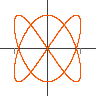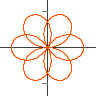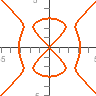Controls Java Version

Jep is a mathematical equation parsing and evaluation library written in java. It features fast evaluation, a configurable architecture allowing custom syntax, support for BigDecimal, complex numbers and vectors It is developed by Singular Systems

GWT-Jep is a port of Jep to run in javascript and has all the features of Jep apart from a small set of changes necessary for javascript compatibility. It is compiled from Java using the Google Web Toolkit. It is available as a seperate package from SingSurf.

The parametrized surfaces application draws surfaces in 3D. It uses the three.js for the 3D graphics. Equations can be specified either as a single height function:

`a x^2+b y^3`

Or as three equations in an array

`[x,x*y,y^2]`

## Jep plotting appsGraphs
`y=a x^2+b x+c`
JavaScript Version
Java VersionParametric
`x=cos(t), y=sin(t)`
JavaScript Version
Java VersionPolar
`r = cos(3 th/2)`
JavaScript Version
Java VersionComplex
`(z+a)/(z+b)`
JavaScript VersionImplicit
`x^2+y^2=1`
JavaScript Version
Java Version
Msqli defined